# Acceleration

### Introduction

Acceleration refers to any change in velocity.
An object moving at a constant velocity will have zero acceleration.
An object moving at a constant speed can still have acceleration if it's changing its direction.
We sometimes refer to a decrease in velocity (a slowing down) as an acceleration.
Acceleration is:
• Rate at which velocity changes
• Vector quantity, with magnitude and direction
The direction of the acceleration vector is that of the change in velocity, Δv.
If velocity is increasing, the acceleration is in the same direction as the velocity as depicted below: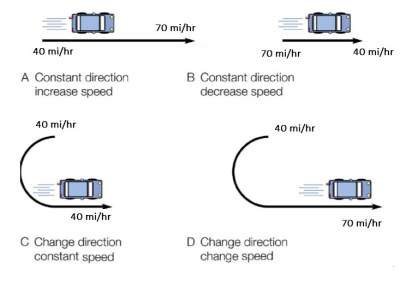How about a car on the circular track having a constant speed (see below), but with a changing velocity every second.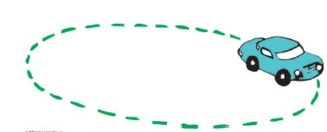Question: Explain why.
Solution:
An object can be moving and still not accelerating.
Acceleration has to do with the change of velocity's direction and/or magnitude.
If an object is not changing its velocity, then the object is not accelerating.

### The Meaning of Constant Acceleration

The data table below shows a car changing its velocity by 15 km/h in each consecutive second,
this mean the car is accelerating.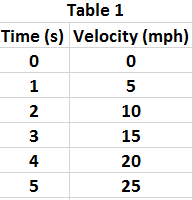As per the above table 1, since the velocity is changing by a constant amount each second,
the acceleration is said to be constant.

#### Examples

Example 1: The two tables below represent accelerating objects: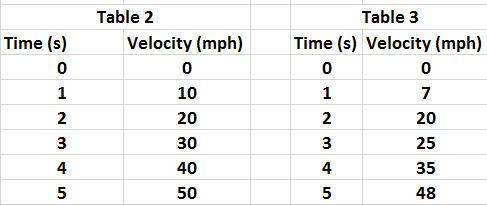Question: which one of them has constant acceleration?

To calculate the acceleration, we need to measure the initial velocity v0 at the beginning of the time interval when t = 0,
and the final velocity of the object vf at the end of the time interval when t = tf.
The Accelaration formula is as follows:
Acceleration a = Change in Velocity / Time Interval = Δv / Δt

Example 2: The car in the figure below moves in constant acceleration from an initial speed of 22 m/s to a final speed of 32 m/s.
If this increase of velocity took 5 seconds, calculate the car acceleration.

Solution:
a = Δv / Δt = 10 m/s / 5 s = 2 m/s2

#### Exercise

Question 1: What is the acceleration of a car that maintains a constant velocity of 120 km/h for 30 s? Answer: Zero

Question 2: Show that the acceleration of a speed boat is 25 m/s2 when it increases its velocity from rest to 50 m/s in 2 s.
Solution:
a = Δv / Δt = 50 m/s / 2 s = 25 m/s2

Challenging Question: Use the example above to calcualte the distance traveled by the speed boat in 4 s.
Answer: d = vi × t + ½ × a × t2
vi is the initial speed and in this case it is equal to zero.
Hence distance = ½×a×t2 = 0.5× 5 × 16 = 40 m.

#### Average Acceleration

A car starting from rest, accelerates to a velocity of 40 m/s due West in a time of 8s as illustrated below:a = (40 m/s) / 8s = (5 m/s)/s = 5 m/s2

### Gravity and Free-Falling Bodies

In the absence of friction, all bodies, large or small, heavy or light,
fall to earth at the same rate with the same acceleration and is called acceleration due to gravity.
Such bodies are in a state of free fall.

Acceleration due to Gravity (g) = 9.80 m/s2
The instantaneous speed (or velocity) v of an object falling from rest after a time t is v = gt
The equation indicates that the speed increases by 9.8 m/s in each second of fall.
The table below shows how the speed (or velocity) changes each second. In here, we rounded off g to 10 m/s2.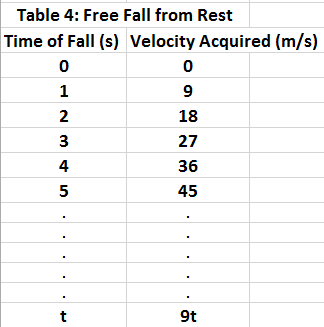### Example

A ball is dropped from a roof of the house which takes 5 seconds to hit the ground.
Question: Calculate the velocity before the ball crashes to the ground. (g=10m/s2)
Solution:
Assume gravity g = 10m/s2
Velocity is: V=g × t = 10m/s2 × 5s=50m/s.